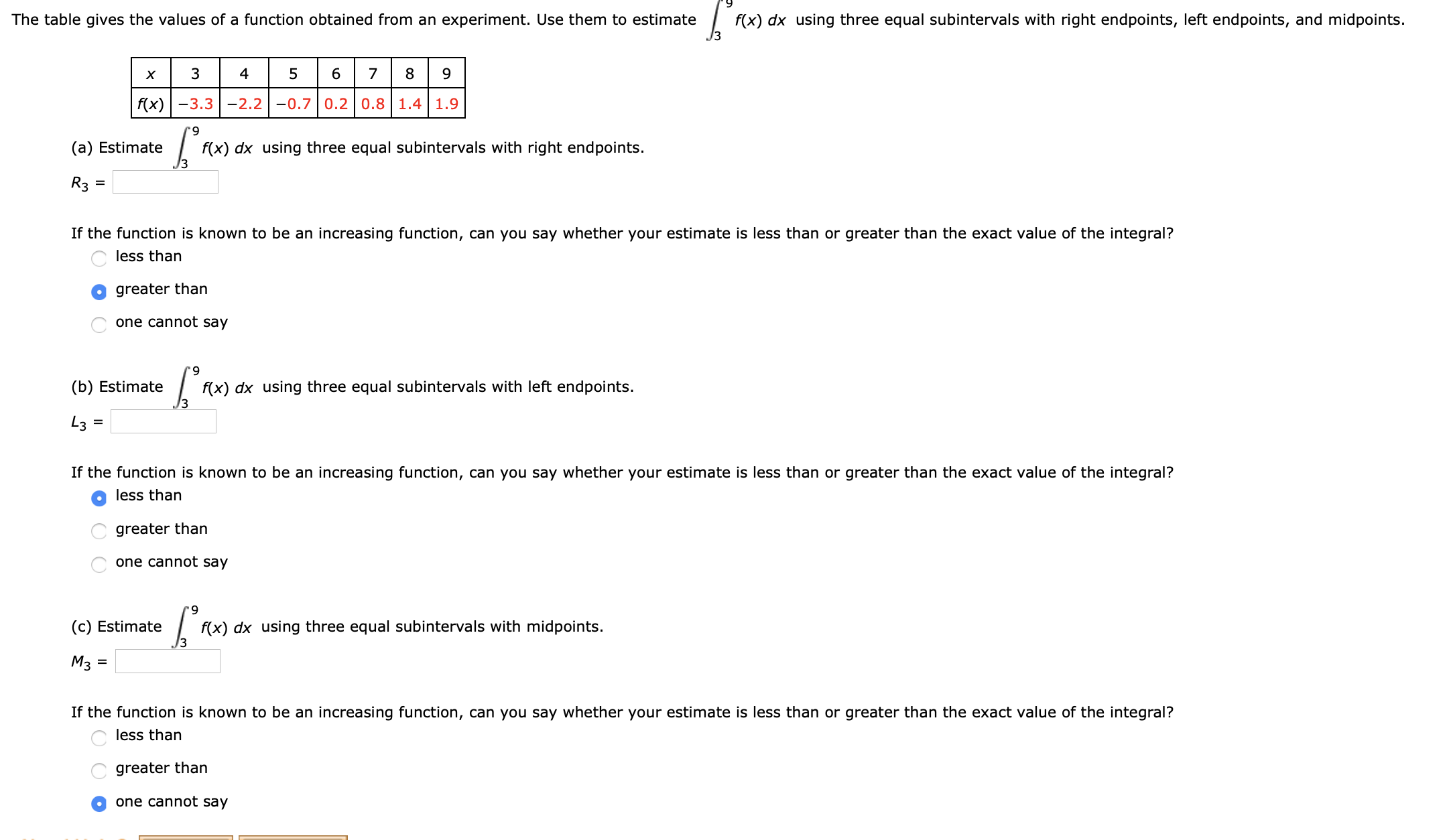# f(x) dx using three equal subintervals with right endpoints, left endpoints, and midpoints.The table gives the valuess of a function obtained from an experiment. Use them to estimate894567хf(x)3.3-2.2-0.7 0.2 0.8 1.4 1.9(a) Estimatef(x) dx using three equal subintervals with right endpoints.R3If the function is known to be an increasing function, can you say whether your estimate is less than or greater than the exact value of the integral?less thangreater thanone cannot say(b) Estimatef(x) dx using three equal subintervals with left endpoints.3L3 =If the function is known to be an increasing function, can you say whether your estimate is less than or greater than the exact value of the integral?O less thangreater thanone cannot say(c) Estimatef(x) dx using three equal subintervals with midpoints.МзIf the function is known to be an increasing function, can you say whether your estimate is less than or greater than the exact value of the integral?less thangreater thanone cannot say

Question
283 viewshelp_outlineImage Transcriptionclosef(x) dx using three equal subintervals with right endpoints, left endpoints, and midpoints. The table gives the valuess of a function obtained from an experiment. Use them to estimate 8 9 4 5 6 7 х f(x)3.3-2.2-0.7 0.2 0.8 1.4 1.9 (a) Estimate f(x) dx using three equal subintervals with right endpoints. R3 If the function is known to be an increasing function, can you say whether your estimate is less than or greater than the exact value of the integral? less than greater than one cannot say (b) Estimate f(x) dx using three equal subintervals with left endpoints. 3 L3 = If the function is known to be an increasing function, can you say whether your estimate is less than or greater than the exact value of the integral? O less than greater than one cannot say (c) Estimate f(x) dx using three equal subintervals with midpoints. Мз If the function is known to be an increasing function, can you say whether your estimate is less than or greater than the exact value of the integral? less than greater than one cannot say fullscreen
check_circle

star
star
star
star
star
1 Rating
Step 1

To determine the integral:

Part a) Using three equal subintervals with right endpoints.

Part b) Using three equal subintervals with left endpoints.

Part c) Using three equal subintervals with midpoints.

Step 2

Given:

Step 3

Formula Used:

...

### Want to see the full answer?

See Solution

#### Want to see this answer and more?

Solutions are written by subject experts who are available 24/7. Questions are typically answered within 1 hour.*

See Solution
*Response times may vary by subject and question.
Tagged in

### Integration